# KSEEB SSLC Class 10 Maths Solutions Chapter 8 Real Numbers Ex 8.4

In this chapter, we provide KSEEB SSLC Class 10 Maths Solutions Solutions Chapter 8 Real Numbers Ex 8.4 for English medium students, Which will very helpful for every student in their exams. Students can download the latest KSEEB SSLC Class 10 Maths Solutions Solutions Chapter 8 Real Numbers Ex 8.4 pdf, free KSEEB SSLC Class 10 Maths Solutions Solutions Chapter 8 Real Numbers Ex 8.4 pdf download. Now you will get step by step solution to each question.

### Karnataka State Syllabus Class 10 Maths SolutionsSolutions Chapter 8 Real Numbers Ex 8.4

Question 1.
Without actually performing the long division, state whether the following rational numbers will have a terminating decimal expansion or a non-terminating repeating decimal expansion :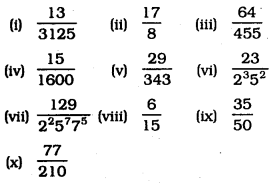Solution:
(i)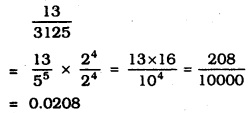∴ This is terminating decimal expansion.

(ii)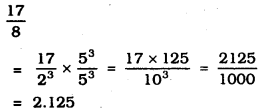∴ This is terminating decimal expansion.

(iii)
64455=265×7×13
∴ This is non-terminating repeating expansion.

(iv)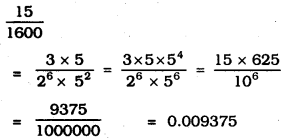∴ This is terminating decimal expansion.

(v)
29343=2973
∴ This is non-terminating repeating expansion.

(vi)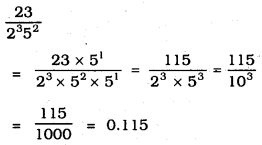∴ This is terminating decimal expansion.

(vii)
129225775
∴ This is non-terminating repeating expansion.

(viii)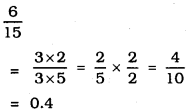∴ This is terminating decimal expansion.

(ix)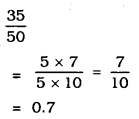∴ This is terminating decimal expansion.

(x)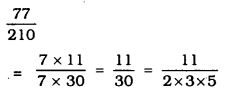∴ This is non-terminating repeating expansion.

Question 2.
Write down the decimal expansions of those rational numbers in Question 1 above which have terminating decimal expansions.
Solution: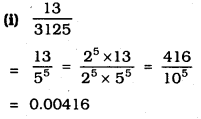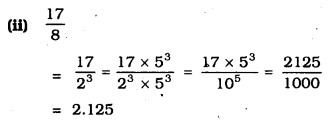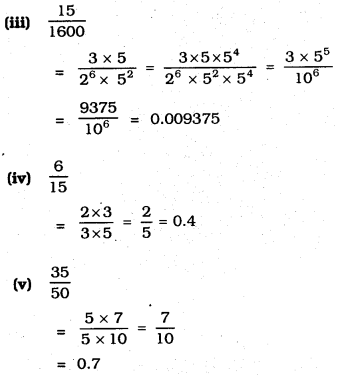Question 3.
The following real numbers have decimal expansions as given below. In each case, decide whether they are rational or not. If they are rational, and of the form pq, what can you say about the prime factors of q?
(i) 43.123456789
(ii) 0.120120012000120000………..
(iii) 43.123456789¯¯¯¯¯¯¯¯¯¯¯¯¯¯¯¯¯¯¯¯¯¯
Solution:
(i) 43.123456789
pq is a rational number
Prime factor of q:
q = 10= 29 × 59

(ii) 0.120120012000120000…………
This is an irrational number

(iii) 43.123456789¯¯¯¯¯¯¯¯¯¯¯¯¯¯¯¯¯¯¯¯¯¯
This is a rational number.
Because, Prime factors of ‘q’ are contrinued in non-terminating decimal expansion.

All Chapter KSEEB Solutions For Class 10 Maths

—————————————————————————–

All Subject KSEEB Solutions For Class 10

*************************************************

I think you got complete solutions for this chapter. If You have any queries regarding this chapter, please comment on the below section our subject teacher will answer you. We tried our best to give complete solutions so you got good marks in your exam.

If these solutions have helped you, you can also share kseebsolutionsfor.com to your friends.

Best of Luck!!